Conditional Probability - Values given

Chapter 13 Class 12 Probability
Concept wise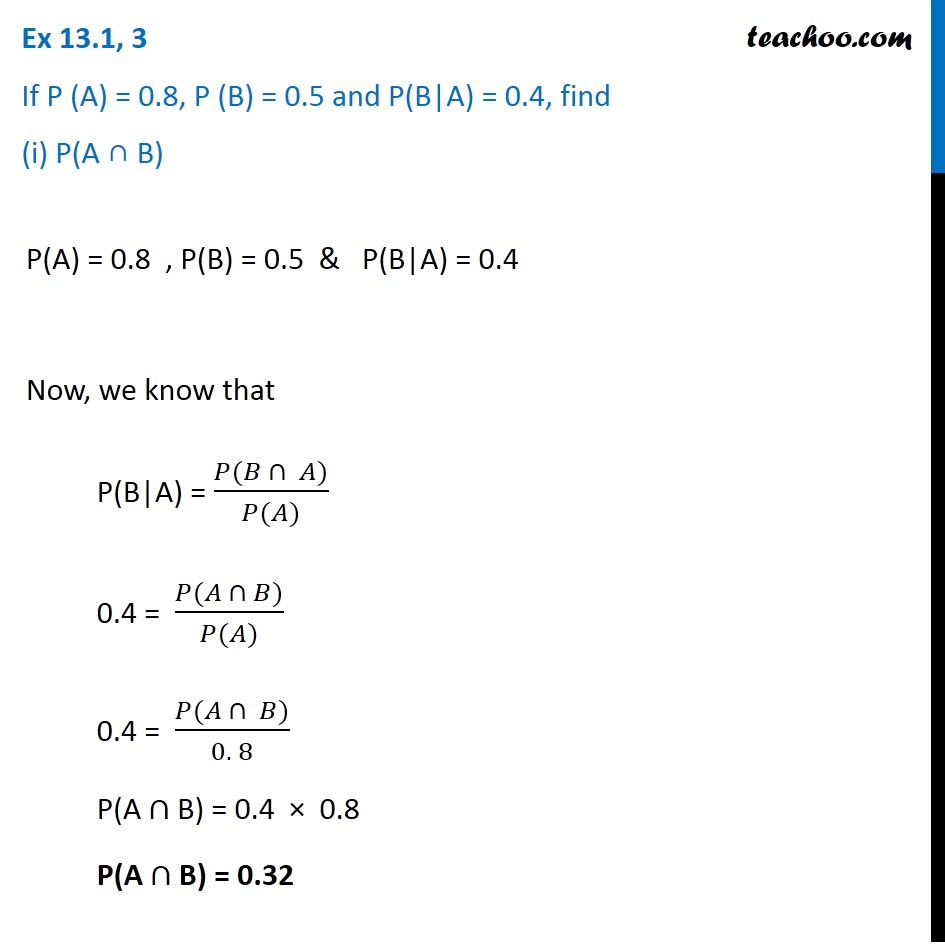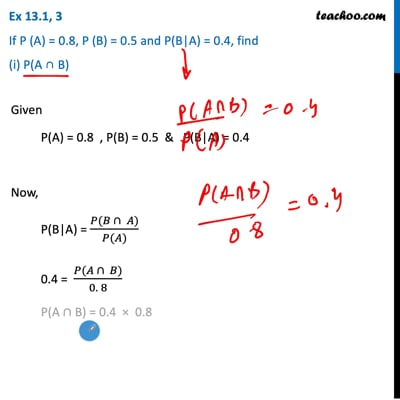This video is only available for Teachoo black users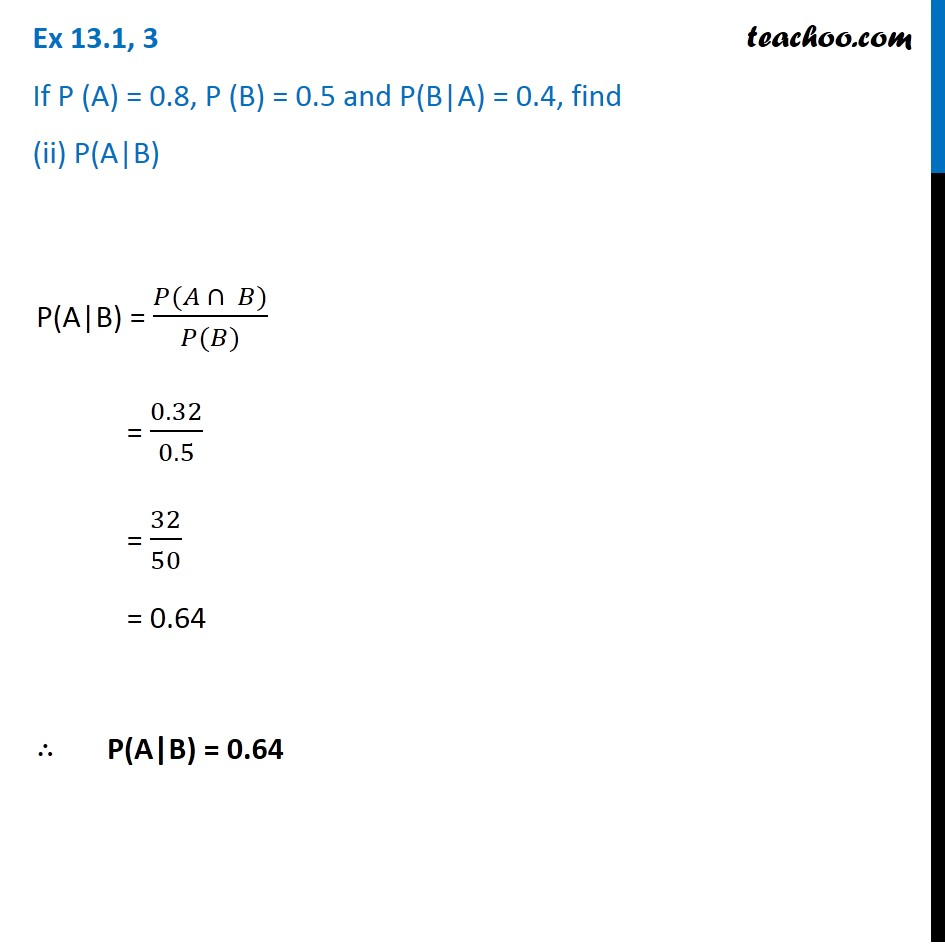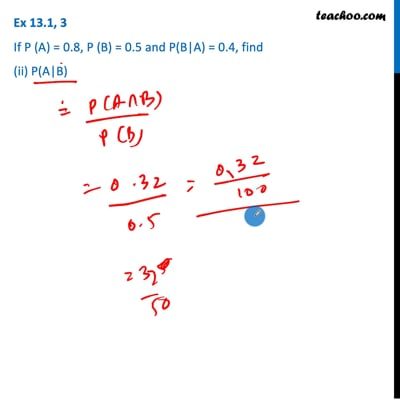This video is only available for Teachoo black users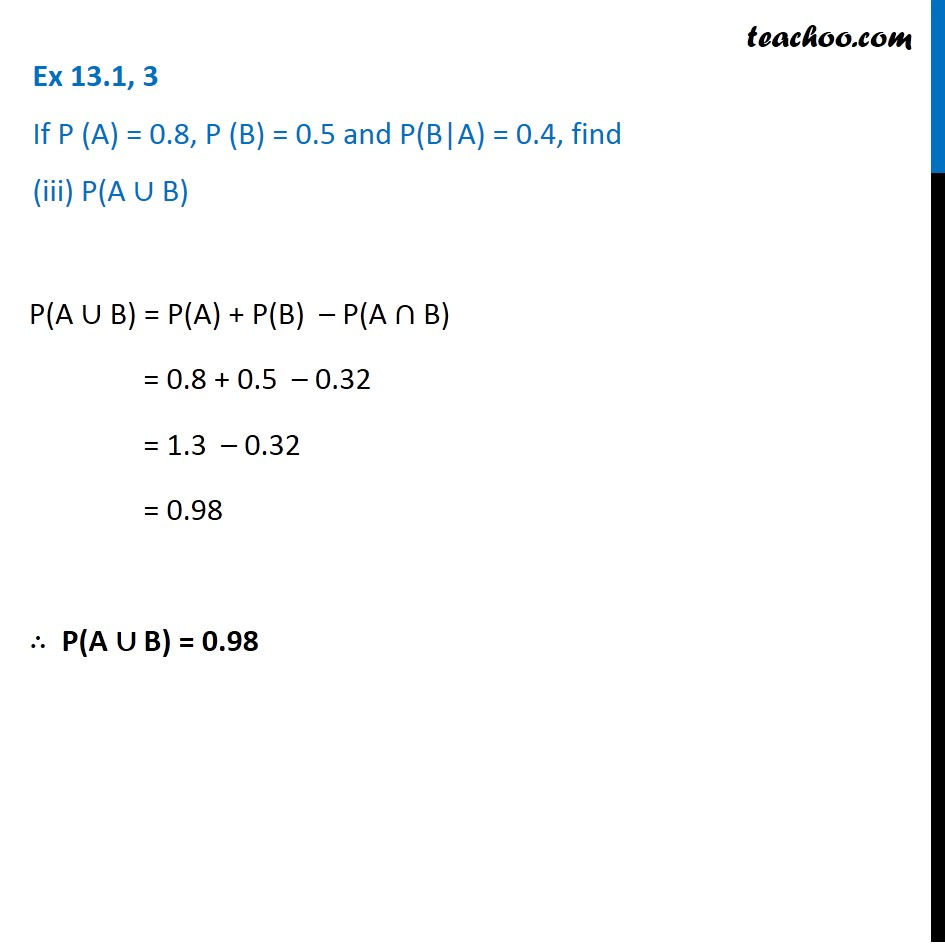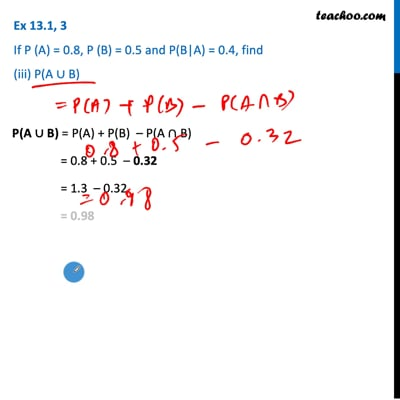This video is only available for Teachoo black users

Introducing your new favourite teacher - Teachoo Black, at only ₹83 per month

### Transcript

Ex 13.1, 3 If P (A) = 0.8, P (B) = 0.5 and P(B|A) = 0.4, find (i) P(A ∩ B)P(A) = 0.8 , P(B) = 0.5 & P(B|A) = 0.4 Now, we know that P(B|A) = (𝑃(𝐵 ∩ 𝐴))/(𝑃(𝐴)) 0.4 = (𝑃(𝐴 ∩ 𝐵))/(𝑃(𝐴)) 0.4 = (𝑃(𝐴 ∩ 𝐵))/(0. 8) P(A ∩ B) = 0.4 × 0.8 P(A ∩ B) = 0.32 Ex 13.1, 3 If P (A) = 0.8, P (B) = 0.5 and P(B|A) = 0.4, find (ii) P(A|B)P(A|B) = (𝑃(𝐴 ∩ 𝐵))/(𝑃(𝐵)) = 0.32/0.5 = 32/50 = 0.64 ∴ P(A|B) = 0.64 Ex 13.1, 3 If P (A) = 0.8, P (B) = 0.5 and P(B|A) = 0.4, find (iii) P(A ∪ B)P(A ∪ B) = P(A) + P(B) – P(A ∩ B) = 0.8 + 0.5 – 0.32 = 1.3 – 0.32 = 0.98 ∴ P(A ∪ B) = 0.98